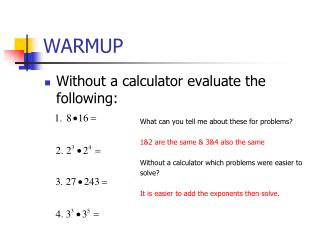Download PresentationWARMUP# WARMUP - PowerPoint PPT Presentation

Download Presentation##### WARMUP

Download Policy: Content on the Website is provided to you AS IS for your information and personal use and may not be sold / licensed / shared on other websites without getting consent from its author. While downloading, if for some reason you are not able to download a presentation, the publisher may have deleted the file from their server.

- - - - - - - - - - - - - - - - - - - - - - - - - - - E N D - - - - - - - - - - - - - - - - - - - - - - - - - - -
##### Presentation Transcript

1. WARMUP • Without a calculator evaluate the following: What can you tell me about these for problems? 1&2 are the same & 3&4 also the same Without a calculator which problems were easier to solve? It is easier to add the exponents then solve.

2. Introduction To Logarithms

3. Logarithms were originally developed to simplify complex arithmetic calculations. They were designed to transform multiplicative processes into additive ones. As seen in our Warmup.

4. If at first this seems like no big deal, then try multiplying 2,234,459,912 and 3,456,234,459. Without a calculator ! Clearly, it is a lot easier to add these two numbers.

5. Today of course we have calculators and scientific notation to deal with such large numbers. So at first glance, it would seem that logarithms have become obsolete.

6. Indeed, they would be obsolete except for one very important property of logarithms. It is called the power property and we will learn about it in another lesson. For now we need only to observe that it is an extremely important part of solving exponential equations.

7. Our first job is to try to make some sense of logarithms.

8. Our first question then must be: What is a logarithm ?

9. Of course logarithms have a precise mathematical definition just like all terms in mathematics. So let’s start with that.

10. Definition of Logarithm Suppose b>0 and b≠1, there is a number ‘p’ such that:

11. Now a mathematician understands exactly what that means. But, many a student is left scratching their head.

12. The first, and perhaps the most important step, in understanding logarithms is to realize that they always relate back to exponential equations.

13. You must be able to convert an exponential equation into logarithmic form and vice versa. So let’s get a lot of practice with this !

14. Example 1: Below is a typical Logarithmic equation. Does it make any sense to you? At this point of course not, but lets rewrite in a form you understand.

15. Now does this make sense to you? Of course! Two to the third power equals eight. Written as a logarithem we simply say: What power does 2 have to be raised to equal 8.

16. CIRCLE OF LOGS Now lets see that again This method was developed by a not so famous Mathematician and he dubbed it the “Circle of Logs”

17. Unfortunately “Circle of Logs” never really caught on but it is an interesting way to remember how to convert from Logarithmic form to exponential form, and visa versa. So that Mathematician (Mr. Eagle) still teaches it to his students. So now you try it on your own.

18. Rewrite the following to exponential form.

19. By the way it works both ways, lets see how it works. Now that is really easy!!!

20. Now you try!

21. Example 1a: Solution: Read as: “the log base 4 of 16 is equal to 2”.

22. Example 1b: Solution:

23. Okay, so now it’s time for you to try some on your own.

24. We now know that a logarithm is perhaps best understood as being closely related to an exponential equation. In fact, whenever we get stuck in the problems that follow we will return to this one simple insight. We might even state a simple rule.

25. When working with logarithms, if ever you get “stuck”, try rewriting the problem in exponential form. Conversely, when working with exponential expressions, if ever you get “stuck”, try rewriting the problem in logarithmic form.

26. Let’s see if this simple rule can help us solve some of the following problems.

27. Solution: Let’s rewrite the problem in exponential form. We’re finished !

28. Solution: Rewrite the problem in exponential form.

29. Example 3 Solution: Try setting this up like this: Now rewrite in exponential form.

30. Example 4 Solution: First, we write the problem with a variable. Now take it out of the logarithmic form and write it in exponential form.